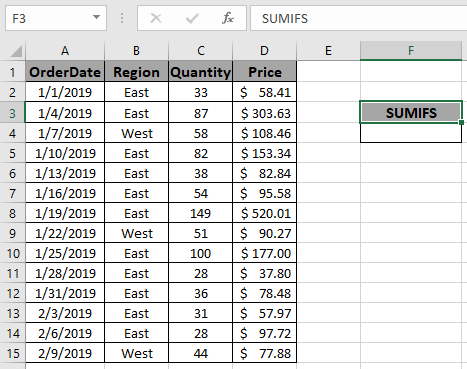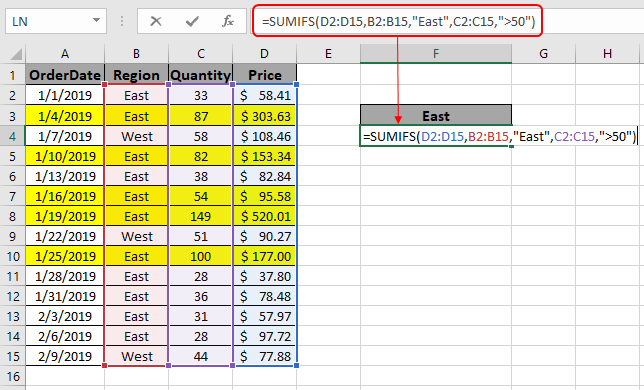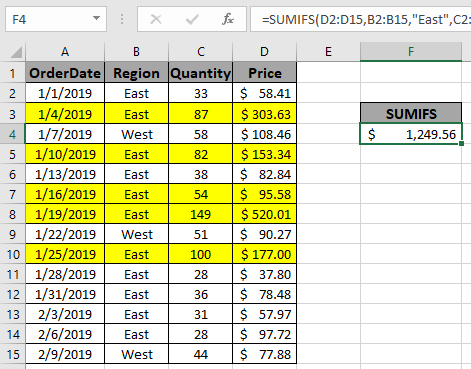# How to Sum With 2 or More Criteria

In this article, we will learn about how to Sum if values having multiple criteria using SUMIFS in Excel.

In simple words, while working with a long data sheet. Sometimes we need to find the find the sum of values having multiple criteria. The SUMIFS function formula finds the sum of the array where multiple conditions need to be satisfied.

The SUMIFS function is used to return the sum of range with multiple criteria.

Syntax of formula:

= SUMIFS ( Sum_range , range1 , "value1" , range2, ">value2", ….)

Sum_range : range where sum is required

range1 : range where criteria value1 is applied

Value1 : value criteria applied on range1

range2 : range where criteria value2 is applied

Value2 : value criteria applied on range2

Let’s sum with 2 criteria using this function.

Example:
Here we have a list of received orders and their respective values.Here we need to find the total price if the region is EAST and Quantity is greater than 50.

We will use the SUMIFS formula to get the total amount:

= SUMIFS ( D2:D15 , B2:B15 , "East" , C2:C15 , ">50" )

D2:D15 : Sum_range are the values that get summed up.

B2:B15 :  range where criteria value1 is applied.

"East" : value criteria applied on range1.

C2:C15 : range where criteria value2 is applied.

">50" : value criteria applied on range2.

Explanation for the formula:

Here is the range B2:B15 checks for the value East (Region) and in the range C2:C15 catches all quantities greater than 50. Then the function finds out the sum from the sum_range (D2:D15) taking the values satisfying both conditions.Here the arguments to the function is given as cell reference.
Use the formula as stated above to calculate the total price and click Enter.As you can see the formula returns \$1249.56 , the Total price for the yellow rows marked.

Notes:

1. The SUMIFS function supports logical operators like <, >, <>, = but these are used using double quote sign ( " ) .
2. The SUMIFS function also supports Wildcards ( * , ? ) which helps in extracting values by having phrases.
3. Non- numeric values must be provided in double quotes ("value").
4. The function returns the sum of the values satisfying all the conditions.

Hope you understand how to get the sum if you have multiple criteria in Excel. Explore more articles in the Excel SUMIFS function here. Please feel free to state your query or feedback for the above article.

Related Articles

3 SUMIF with Or Formulas

How to Use SUMIFS Function in Excel

SUMIFS using AND-OR logic

SUMIF with non-blank cells

SUMIFS with dates in Excel

Popular Articles

Edit a dropdown list

If with conditional formatting

If with wildcards

Vlookup by date

Terms and Conditions of use

The applications/code on this site are distributed as is and without warranties or liability. In no event shall the owner of the copyrights, or the authors of the applications/code be liable for any loss of profit, any problems or any damage resulting from the use or evaluation of the applications/code.